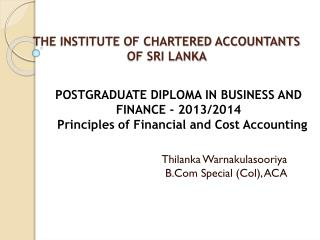DownloadDownload PresentationTHE INSTITUTE OF CHARTERED ACCOUNTANTS OF SRI LANKA

# THE INSTITUTE OF CHARTERED ACCOUNTANTS OF SRI LANKA

Download Presentation## THE INSTITUTE OF CHARTERED ACCOUNTANTS OF SRI LANKA

- - - - - - - - - - - - - - - - - - - - - - - - - - - E N D - - - - - - - - - - - - - - - - - - - - - - - - - - -
##### Presentation Transcript

1. THE INSTITUTE OF CHARTERED ACCOUNTANTS OF SRI LANKA Thilanka WarnakulasooriyaB.Com Special (Col), ACA POSTGRADUATE DIPLOMA IN BUSINESS AND FINANCE - 2013/2014Principles of Financial and Cost Accounting

2. Principles of Cost Accounting Cost Accounting A method of accounting in which all costs incurred in carrying out an activity or accomplishing a purpose are collected, classified, and recorded. This data is then summarized and analyzed to arrive at a selling price, or to determine where savings are possible.

3. Cost “ The amount of expenditure incurred or attributable to a specified thing or activity” i.e Product “A” cost is Rs. 500 Cost object A Product, Service, Center activity, Customer or distribution channel in relation to which cost are ascertained. • i.e. XYZ company produces a line of 10-speed bicycles which is a cost object in their product line.

4. Cost Center It is a location, activity, item or function of an organization in respect of which costs are ascertained Examples of cost centers include research and development departments, marketing departments, help desks and customer service

5. Cost Classification • According to the elements • According to the purpose • According to the functions • According to the Behavior

6. According to purpose The cost are classified according to the reason for which they have been incurred. Accordingly it can be divided in to two. • Direct Cost • Cost that can be directly identified with the cost unit/Object • i.e. Direct Material, Direct Labor, Direct Expenses • Indirect cost • Cost that can not be directly identified with the cost unit/Object ( all indirect cost called as overheads) • i.e. indirect Material, indirect Labor, indirect Expenses

7. i. e Cost classification of a Garment

8. According to Behavior Costs can be classified into variable, fixed, semi-variable, or step-costs according to how they behave with respect of changes in activity levels i.e. Fixed cost Variable cost Semi Variable cost

9. Fixed Cost A cost remains constant over a relevant range of activity level. However, unit fixed cost falls with an increase in activity volume. i.e. Rent, Depreciation, Salaries of Executives. ABC Company total fixed cost per month is Rs.500,000

10. Graphical Representation-Total Fixed cost

11. Fixed cost per unit • Fixed cost per unit means the total fixed cost divided by number of units.

12. Variable cost • Cost increases or decreases in direct proportion to levels of activity, • However the unit variable cost remains constant • i.e. Raw material cost, Direct labor costs necessary to complete a certain project.

13. Step cost It remains constant for a range of activity levels, then, on further increase in activity, the cost jumps to a new level and remains constant over a certain range until the next jump occurs.

14. Total Cost Total of Variable cost & Fixed cost Accordingly Total Cost = Total Fixed Cost + Total Variable Cost Total Cost = Total Fixed Cost + (Variable Cost per ) unit X no Units

15. ABC Ltd is producing product “A” & Management Accountant has found following information • Rent of the Factory 100,000 • Salaries of Executive staff 250,000 • Plant Depreciation 50,000 He also found following information with regard to per unit of product “A” Direct Martial used : 3Kg @ Rs. 20 Direct Labor : 2 Hrs @ Rs. 50 Per Hour Royalty fee : 100 Per unit Find out total cost of producing 0 units 5,000 units 10,000 Units

16. Semi-variable cost It processes characteristics of both fixed and variable cost It increases or decreases with activity level but not in direct proportion ( also called mixed cost)

17. Cost Estimation:Determine the cost behavior according to the level of activity • High Low Method : 1st Identify the Variable cost & then it used to ascertain the fixed cost Steps • Based on a table of total costs and activity levels, determine the high and low activity levels. 2.Calculate the variable cost per unit using high low activity level Variable cost per unit = Change in the total cost Change in the activity Level 3.Use following equation to determine the fixed cost by substituting per unit variable cost Fixed Cost= Fixed Cost +( Variable cost per unit X No of units)

18. Ex:01 • Calculate the cost of producing 200 units